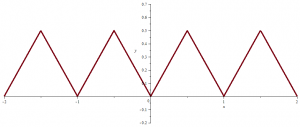# A field that can be ordered in two distinct ways

For a short reminder about ordered fields you can have a look to following post. We prove there that $$\mathbb{Q}$$ can be ordered in only one way.

That is also the case of $$\mathbb{R}$$ as $$\mathbb{R}$$ is a real-closed field. And one can prove that the only possible positive cone of a real-closed field is the subset of squares.

However $$\mathbb{Q}(\sqrt{2})$$ is a subfield of $$\mathbb{R}$$ that can be ordered in two distinct ways. Continue reading A field that can be ordered in two distinct ways

# An infinite field that cannot be ordered

## Introduction to ordered fields

Let $$K$$ be a field. An ordering of $$K$$ is a subset $$P$$ of $$K$$ having the following properties:

ORD 1
Given $$x \in K$$, we have either $$x \in P$$, or $$x=0$$, or $$-x \in P$$, and these three possibilities are mutually exclusive. In other words, $$K$$ is the disjoint union of $$P$$, $$\{0\}$$, and $$-P$$.
ORD 2
If $$x, y \in P$$, then $$x+y$$ and $$xy \in P$$.

We shall also say that $$K$$ is ordered by $$P$$, and we call $$P$$ the set of positive elements. Continue reading An infinite field that cannot be ordered

# A function that is everywhere continuous and nowhere differentiable

Let $$f_1(x) = |x|$$ for $$| x | \le \frac{1}{2}$$, and let $$f_1$$ be defined for other values of $$x$$ by periodic continuation with period $$1$$. $$f_1$$ graph looks like following picture:$$f_1$$ is continuous everywhere and differentiable on $$\mathbb{R} \setminus \mathbb{Z}$$. Continue reading A function that is everywhere continuous and nowhere differentiable

# A compact convex set whose extreme points set is not close

Let’s remind that an extreme point $$c$$ of a convex set $$C$$ in a real vector space $$E$$ is a point in $$C$$ which does not lie in any open line segment joining two points of $$C$$.

## The specific case of dimension $$2$$

Proposition: when $$C$$ is closed and its dimension is equal to $$2$$, the set $$\hat{C}$$ of its extreme points is closed.
Continue reading A compact convex set whose extreme points set is not close

# An unbounded positive continuous function with finite integral

Consider the piecewise linear function $$f$$ defined on $$[0,+\infty)$$ taking following values for all $$n \in \mathbb{N^*}$$:
$f(x)= \left\{ \begin{array}{ll} 0 & \mbox{if } x=0\\ 0 & \mbox{if } x=n-\frac{1}{2n^3}\\ n & \mbox{if } x=n\\ 0 & \mbox{if } x=n+\frac{1}{2n^3}\\ \end{array} \right.$

The graph of $$f$$ can be visualized in the featured image of the post. Continue reading An unbounded positive continuous function with finite integral